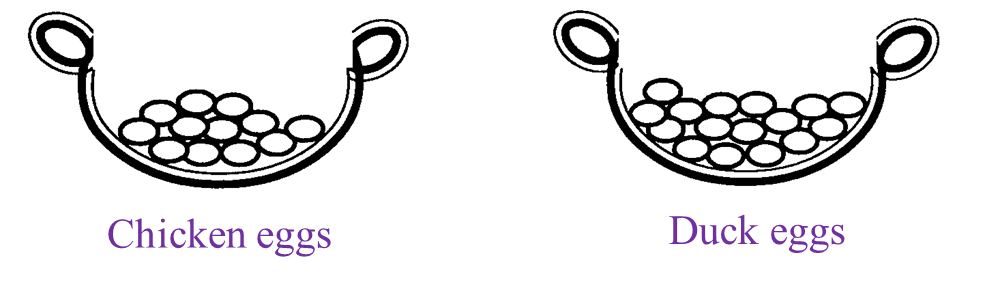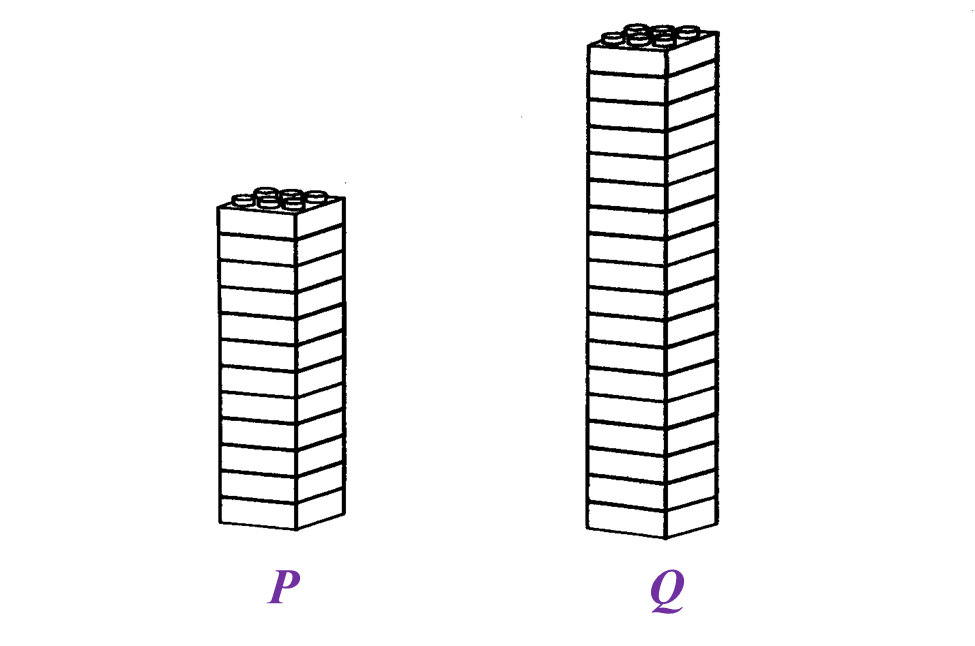# 5.2.3 Ratio, Rates and Proportions (I), PT3 Focus Practice

Question 11:
Diagram below shows two baskets of eggs.(a) State the ratio of the number of chicken eggs to the number of duck eggs.
(b) A number of eggs need to be added into each basket so that the ratio in (a) remain unchanged. Find the minimum number of eggs to be added in each basket.

Solution
(a)
Ratio of the number of chicken eggs to the number of duck eggs
= 12 : 16
= 3 : 4

(b)
Let the number of eggs need to be added into each basket = n
= 3n + 4n

Minimum value of n = 1
Hence, the minimum number of eggs to be added into each basket
= 3(1) + 4(1)
= 3 + 4
= 7 (3 chicken eggs and 4 duck eggs need to be added to each basket)

Question 12:
Diagram below shows two baskets, A and B, filled with candies.(a) State the ratio of the number of candies in basket A to the number of candies in basket B.
(b) If 20 candies are added into basket A, calculate the number of candies which need to be added into basket B so that the ratio in (a) remain unchanged.

Solution
(a)
Ratio of the number of candies in basket A to the number of candies in basket B
= 10 : 12
= 5 : 6

(b)
If 20 candies are added into basket A, the number of candies which need to be added into basket B so that the ratio 5 : 6 remain unchanged
= 20 ÷ 5 × 6
= 4 × 6
= 24

Question 13:
Karim built two towers using toy bricks of the same size as shown in Diagram below.(a) State the ratio of the number of toy bricks in Tower P to the number of toy bricks in Tower Q.
(b) A number of toy bricks need to be removed from each tower so that the ratio in (a) remains unchanged.
Find the total number of toy bricks to be removed from each tower.

Solution
(a)
Tower P : Tower Q
= 12 : 18
= 2 : 3

(b)
Let number of toy bricks to be removed from each tower = n
Total number of bricks to be removed
= 2n + 3n
2(1) + 3(1) = 2 + 3 = 5 → (2 bricks from tower P, 3 bricks from tower Q)
2(2) + 3(2) = 4 + 6 = 10 → (4 bricks from tower P, 6 bricks from tower Q)
2(3) + 3(3) = 6 + 9 = 15 → (6 bricks from tower P, 9 bricks from tower Q)
2(4) + 3(4) = 8 + 12 = 20 → (8 bricks from tower P, 12 bricks from tower Q)
2(5) + 3(5) = 10 + 15 = 25 → (10 bricks from tower P, 15 bricks from tower Q)

# 5.2.2 Ratio, Rates and Proportions (I), PT3 Focus Practice

Question 6:
In a campaign of selling T shirt for Merdeka Day makes an amount of profit, RM x. The profit is divided between Jamal and Rafizi in the ratio of 5 : 3. Jamal receives RM 5400 more than Rafizi. Calculate the value of x.

Solution:
Portion of profit for Jamal = ⅝
Portion of profit for Rafizi = ⅜
Given Jamal receives RM 5400 more than Rafizi,

Question 7:
Karim and Roger share RM300 in the ratio of 2 : 3. Karim gives ⅓ of his share to Mandeep. Then Mandeep received RM60 from Roger.
Find the ratio of Karim’s money to roger’s money to Mandeep’s money.

Solution:

Question 8:
Liza received three types of coloured marbles, red, green and yellow in the ratio of 2 : 5 : x. Given that the number of yellow marbles are more than the number of red marbles but less than the number of green marbles.
Calculate the number of yellow marbles that Liza received if the total number of marbles is 120.

Solution:
red : green : yellow = 2 : 5 : x
Given 2 < x < 5, so possible value of x is 3 in order to get a round number from the total of 120 marbles.

Question 9:
John and Mahmud are required to draw a triangle ABC. The ratio of ∠A : ∠B : ∠C of the triangle drawn by John is 4 : 8 : 3 while the triangle drawn by Mahmud is 5 : 5 : 8.
Find the difference between the value of ∠B drawn by John and Mahmud.

Solution:

Question 10:
The number of workers in an office building is 168 and they are placed in level two and level three. The number of workers in level three is 72.
(a) The ratio of workers in level two to level three is x : 3.
Find the value of x.
(b) 162 new workers have just started working in the office building. 3 of them are placed in level two, 93 in level three, while the rest is placed in level four.
Determine the ratio, in the lowest term of the workers of level two to three to four.

Solution:

# 5.2.1 Ratio, Rates and Proportions (I), PT3 Focus Practice

5.2.1 Ratio, Rates and Proportions (I), PT3 Focus Practice

Question 1:
Given x : y = 4 : 5 and x + y = 180. Find the value of x.

Solution:

Question 2:
Given x : y = 9 : 5 and xy = 16. Find the value of x + y.

Solution:

Question 3:
Amy, Rizal and Muthu donated to a charity fund in the ratio of 2 : 1 : 3. The total donation from Amy and Muthu was RM360.
Calculate the amount donated by Rizal.

Solution:
Amy : Rizal : Muthu = 2 : 1 : 3

Question 4:
In the figure, TU: UV : VW = 5 : 7 : 2.

If TU = 30 cm, what is the length of TW?

Solution:

Question 5:
In diagram below, ABC is a triangle.
The sides of the triangle are in the ratio AB : BC: CA = 4 : 7 : 6.
Find the difference in length, in cm, between AB and CA.

Solution:
$\begin{array}{l}AB:BC:CA=4:7:6\\ BC=35cm\\ \\ \frac{AB}{BC}=\frac{4}{7}\\ \frac{AB}{35}=\frac{4}{7}\\ AB=\frac{4}{7}×35\\ \text{}=20cm\\ \frac{CA}{35}=\frac{6}{7}\\ CA=\frac{6}{7}×35\\ \text{}=30cm\end{array}$

Difference in length between AB and CA
= 30 – 20
= 10 cm

# 3.4.1 The Effects of Environmental Pollution (Structured Questions)

Question 1:
Diagram 1.1 shows a type of an environmental pollution in an industrial area.(a)(i) Based on Diagram 1.1, what is released by the factory? [1 mark]

(a)(ii) State the type of pollution based on the answer in (a)(i). [1 mark]

(b) State the effect of pollution shown in Diagram 1.1 on human being. [1 mark]

(c) Suggest one method to control the pollution shown in Diagram 1.1. [1 mark]

(d) Diagram 1.2 shows the effect of environmental pollution due to the heat trapped by gas P.(i) Name gas P. [1 mark]
(ii) Name the phenomenon shown in Diagram 1.2. [1 mark]

(a)(i)
Thick black smoke (soot)

(a)(ii) Air pollution

(b)
The acidic gases in the smoke irritate and corrode the respiratory passage (causing asthma and bronchitis)

(c)
Fit electrostatic precipitators on the chimneys to attract the soot to the inner wall of the chimneys.

(d)(i)
Carbon dioxide

(d)(ii)
Global warming/ Greenhouse effect

Question 2:
Diagram 2 shows the atmospheric layer around the Earth.(a)(i) What is layer K? [1 mark]

(ii) State the importance of layer K. [1 mark]

(b)(i) Name the substance which can damage layer K. [1 mark]

(ii) Name one appliance that releases the substance in (b)(i). [1 mark]

(c) State two effects if layer K is damaged. [2 marks]

(a)(i)
Ozone layer

(a)(ii)
Absorbs harmful ultraviolet rays in sunlight

(b)(i)
Chlorofluorocarbon (CFC)

(b)(ii)
Aerosol spray

(c)
1. Ultraviolet ray reaching the Earth can cause skin cancer and cataract
2. Ultraviolet ray lower our body’s immunity to diseases.

# 4.2.1 Linear Equations I, PT3 Practice 1

4.2.1 Linear Equations I, PT3 Practice 1
Question 1:
Solve the following linear equations.
(a)  4 – 3n = 5n – 4
(b) $\frac{4m-2}{10+m}=\frac{1}{2}$

Solution:
(a)
4 – 3n = 5n – 4
–3n –5n = – 4 – 4
–8n = – 8
8n = 8
n = 8/8 = 1

$\begin{array}{l}\text{(b)}\frac{4m-2}{10+m}=\frac{1}{2}\\ \text{}2\left(4m-2\right)=10+m\\ \text{}8m-4=10+m\\ \text{}8m-m=10+4\\ \text{7}m=14\\ \text{}m=\frac{14}{7}\\ \text{}m=2\end{array}$

Question 2:
Solve the following linear equations.
$\begin{array}{l}\text{(a)}\frac{x}{3}=4-x\\ \text{(b)}\frac{3\left(x-2\right)}{5}=9\end{array}$

Solution:
$\begin{array}{l}\text{(a)}\frac{x}{3}=4-x\\ \text{}x=3\left(4-x\right)\\ \text{}x=12-3x\\ x+3x=12\\ \text{}4x=12\\ \text{}x=\frac{12}{4}\\ \text{}x=3\end{array}$

$\begin{array}{l}\text{(b)}\frac{3\left(x-2\right)}{5}=9\\ \text{}3\left(x-2\right)=9×5\\ \text{}3x-6=45\\ \text{}3x=45+6\\ \text{}3x=51\\ \text{}x=\frac{51}{3}\\ \text{}x=17\end{array}$

Question 3:
Solve the following linear equations.
$\begin{array}{l}\text{(a)}\frac{3m}{4}+15=9\\ \text{(b)}2m+8=3\left(m-2\right)\end{array}$

Solution:
$\begin{array}{l}\text{(a)}\frac{3m}{4}+15=9\\ \text{}\frac{3m}{4}=9-15\\ \text{}\frac{3m}{4}=-6\\ \text{}3m=-6×4\\ \text{}3m=-24\\ \text{}m=\frac{-24}{3}\\ \text{}m=-8\end{array}$

$\begin{array}{l}\text{(b)}2m+8=3\left(m-2\right)\\ \text{}2m+8=3m-6\\ \text{}2m-3m=-6-8\\ \text{}-m=-14\\ \text{}m=14\end{array}$

Question 4:
Solve the following linear equations.
$\begin{array}{l}\text{(a)}11+\frac{2x}{3}=9\\ \text{(b)}\frac{x-5}{3}=\frac{x}{6}\end{array}$

Solution:
$\begin{array}{l}\text{(a)}11+\frac{2x}{3}=9\\ \text{}\frac{2x}{3}=9-11\\ \text{}\frac{2x}{3}=-2\\ \text{}2x=-6\\ \text{}x=\frac{-6}{2}\\ \text{}x=-3\end{array}$

$\begin{array}{l}\text{(b)}\frac{x-5}{3}=\frac{x}{6}\\ \text{}6\left(x-5\right)=3x\\ \text{}6x-30=3x\\ \text{}6x-3x=30\\ \text{}3x=30\\ \text{}x=10\end{array}$

Question 5:
Solve the following linear equations.
$\begin{array}{l}\text{(a) 4}\left(2x-3\right)=24\\ \text{(b)}\frac{y}{2}-\frac{y+4}{3}=5\end{array}$

Solution:

$\begin{array}{l}\text{(a) 4}\left(2x-3\right)=24\\ \text{}8x-12=24\\ \text{}8x=24+12\\ \text{}8x=36\\ \text{}x=\frac{36}{8}\\ \text{}x=4\frac{1}{2}\end{array}$

$\begin{array}{l}\text{(b)}\frac{y}{2}-\frac{y+4}{3}=5\\ \text{}\frac{y×3}{2×3}-\frac{2\left(y+4\right)}{3×2}=5\\ \text{}\frac{3y-2\left(y+4\right)}{6}=5\\ \text{}3y-2y-8=30\\ \text{}y=30+8\\ \text{}y=38\end{array}$

# 4.1 Linear Equations I

4.1 Linear Equations I

4.1.1 Equality
1. An equation is a mathematical statement that joins two equal quantities together by an equality sign ‘=’.
Example: km = 1000 m

2.
If two quantities are unequal, the symbol ‘≠’ (is not equal) is used.
Example: 9 ÷ 4 ≠ 3

4.1.2 Linear Equations in One Unknown
1. A linear algebraic term is a term with one unknown and the power of unknown is one.
Example: 8x, -7y, 0.5y, 3a, …..

2.
A linear algebraic expression contains two or more linear algebraic terms which are joined by a plus or minus sign.
Example:
3x – 4y, 4+ 9, 6x – 2y + 5, ……

3.
A linear equation is an equation involving numbers and linear algebraic terms.
Example:
5x – 4 = 11, 4x + 7 = 15, 3y – 2 = 7

4.1.3 Solutions of Linear Equations in One Unknown
1. Solving an equation is a process of finding the values of the unknown in the equation.
2. The number that satisfies the equation is called the solution or root of the equation.
Example 1:
+ 4 = 12
x = 12 – 4 ← (When +4 is moved to the right of the equation, it becomes –4)
= 8

Example 2:
– 7 = 11
x = 11 + 7 ← (When –7 is moved to the right of the equation, it becomes +7)
= 18

Example
3:

$\begin{array}{l}8x=16\\ x=\frac{16}{8}←\overline{)\begin{array}{l}\text{when the multiplier 8 is moved}\\ \text{to the right of the equation, it}\\ \text{becomes the divisor 8}\text{.}\end{array}}\\ x=2\end{array}$

Example
4:
$\begin{array}{l}\frac{x}{5}=3\\ x=3×5←\overline{)\begin{array}{l}\text{the divisor 5 becomes the}\\ \text{multiplier 5 when moved}\\ \text{to the right of the equation}\text{.}\end{array}}\\ x=15\end{array}$